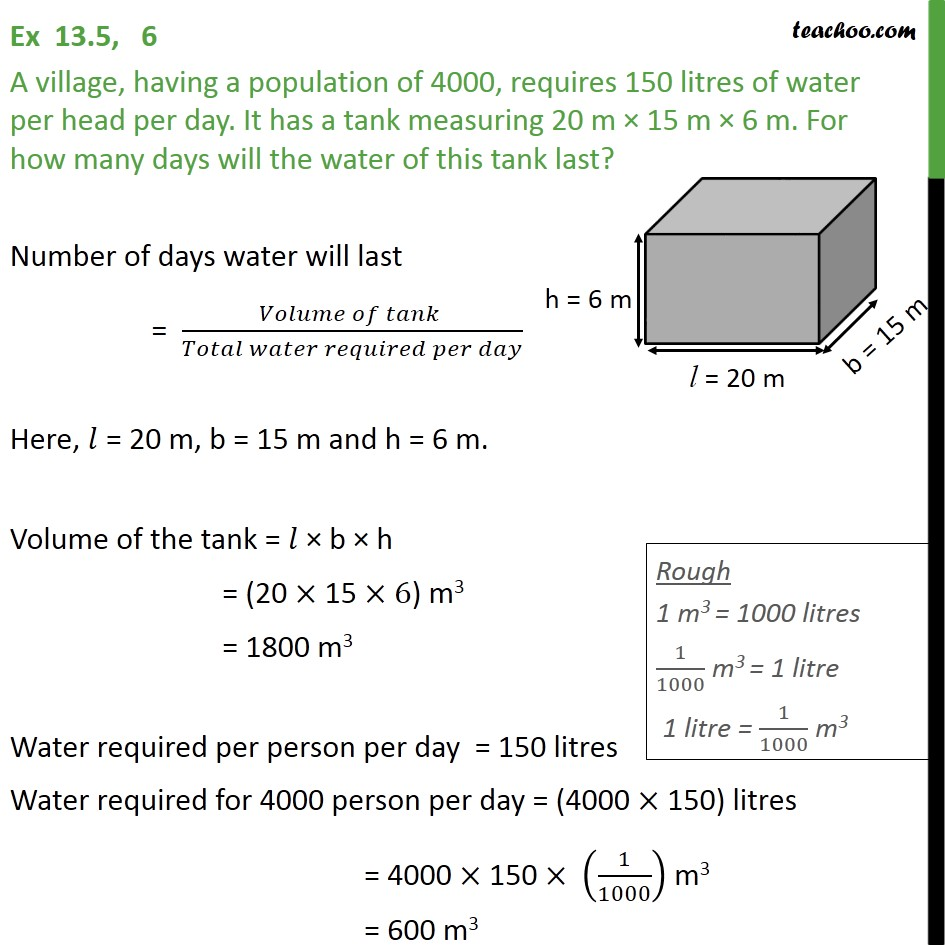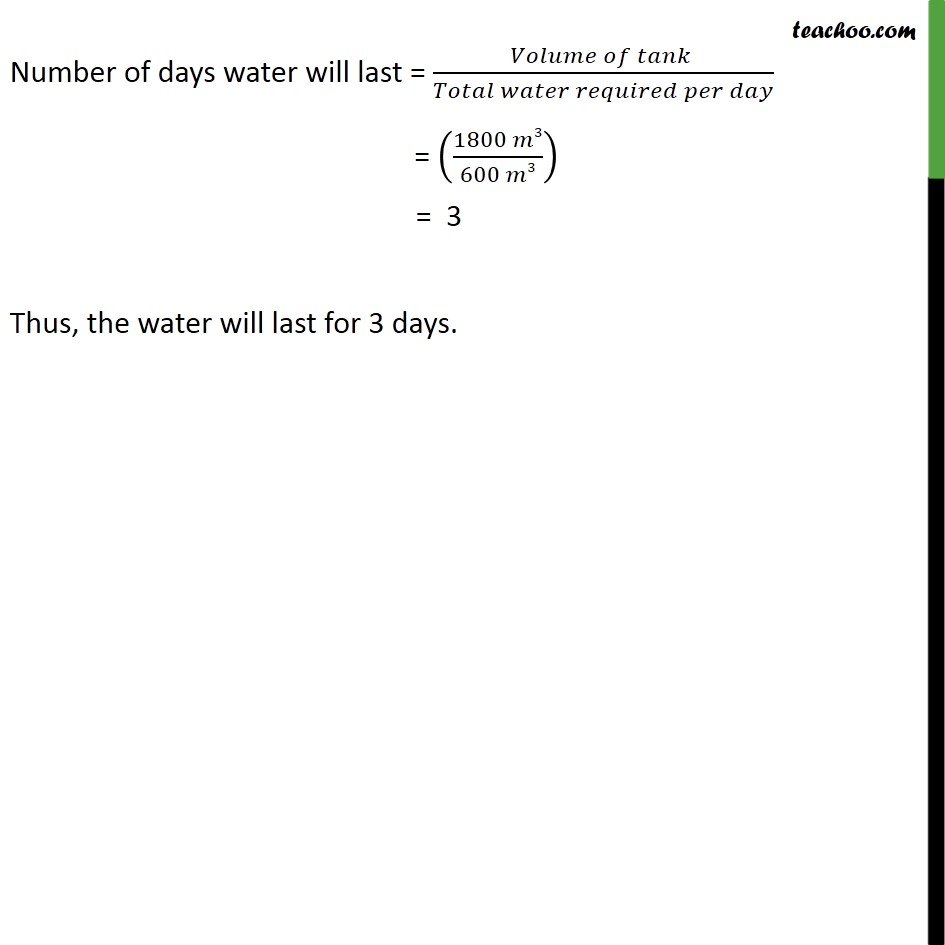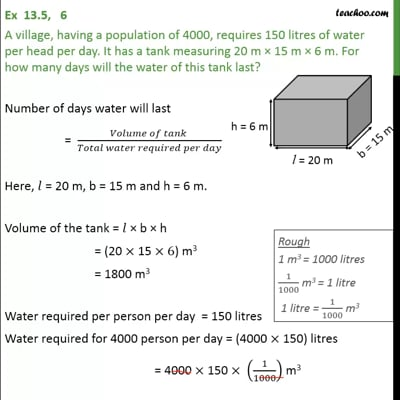Ex 13.5

Chapter 13 Class 9 Surface Areas and Volumes
Serial order wiseThis video is only available for Teachoo black users

Maths Crash Course - Live lectures + all videos + Real time Doubt solving!

### Transcript

Ex 13.5, 6 A village, having a population of 4000, requires 150 litres of water per head per day. It has a tank measuring 20 m × 15 m × 6 m. For how many days will the water of this tank last? Number of days water will last = (𝑉𝑜𝑙𝑢𝑚𝑒 𝑜𝑓 𝑡𝑎𝑛𝑘 )/(𝑇𝑜𝑡𝑎𝑙 𝑤𝑎𝑡𝑒𝑟 𝑟𝑒𝑞𝑢𝑖𝑟𝑒𝑑 𝑝𝑒𝑟 𝑑𝑎𝑦) Here, 𝑙 = 20 m, b = 15 m and h = 6 m. Volume of the tank = 𝑙 × b × h = (20 × 15 ×6) m3 = 1800 m3 Water required per person per day = 150 litres Water required for 4000 person per day = (4000 × 150) litres = 4000 × 150 × (1/1000) m3 = 600 m3 Number of days water will last = (𝐶𝑎𝑝𝑎𝑐𝑖𝑡𝑦 𝑜𝑓 𝑡𝑎𝑛𝑘 )/(𝑡𝑜𝑡𝑎𝑙 𝑤𝑎𝑡𝑒𝑟 𝑟𝑒𝑞𝑢𝑖𝑟𝑒𝑑 𝑝𝑒𝑟 𝑑𝑎𝑦) = ((1800 𝑚3)/(600 𝑚3)) = 30 Thus, the water will last for 30 days. Number of days water will last = (𝑉𝑜𝑙𝑢𝑚𝑒 𝑜𝑓 𝑡𝑎𝑛𝑘 )/(𝑇𝑜𝑡𝑎𝑙 𝑤𝑎𝑡𝑒𝑟 𝑟𝑒𝑞𝑢𝑖𝑟𝑒𝑑 𝑝𝑒𝑟 𝑑𝑎𝑦) = ((1800 𝑚3)/(600 𝑚3)) = 3 Thus, the water will last for 3 days.